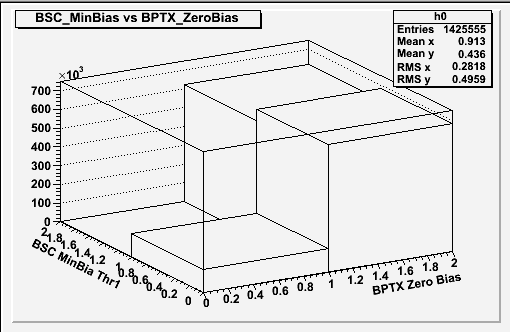# TH2F and GetBinContent(bin)

Hi. I almost feel embarrassed posting this as it should be an easy thing to do!
I have two triggers A and B. Each can be 0 or 1.
I have made a TH2F 2D lego plot from the comparison of these triggers:
MyNtuple->Draw(“A:B” ,"","Lego);
I get a lego plot of 4 ‘cubes’ showing the quantities of (A & B), (A & !B), (!A & B) and (!A & !B) as expected.
I want to get the Numerical values for each of these ‘cubes’. ie. I want to h0->GetBinContents()
but I can’t. Here’s a snippet of the code:

``````...
//Define histogram.
TH2F *h0 = new TH2F("h0","Trigger_Stuff", 2, 0., 2., 2, 0., 2.);
//Histogram two triggers in to h0.
MyNtuple->Draw("TriggerA:TriggerB>>h0","","LEGO");

//Get 'linearized' bin number
int bin = h0->GetBin(0,0);     //Have also tried GetBin(0,0,0)
double trueNeg = h0->GetBinContent(bin);

int bin = h0->GetBin(0,1);     // Have also tried GetBin(0,1,0)
double falseNeg = h0->GetBinContent(bin);

cout << "True Negative:  " << trueNeg << endl;
cout << "False Negative: " << falseNeg << endl;
...

``````

I am receiving some numbers, but they are wrong.
Please let me know if you require the complete code in order to help.

Ultimately, I want to calculate the number of true positive, true negative, false positive and false negative occurrences of trigger A w.r.t Trigger B.
Any help is greatly appreciated. Thanks.
Alan.

you can do directly:

```double trueNeg = h0->GetBinContent(i,j); ```
Rene

Hi Rene.
I should have mentioned that I had already tried this method, as in the following code example. I’ve included the actual TH2 histo declaration and the line that drags the data from the nTuple, just incase I am doing something wrong here.

``````//create TH2D object
TH2D *h0 = new TH2D("h0","MinBias",2, 0., 2.,  2, 0., 2.);
...
//Draw data from nTuple, newHltTree.
newHltTree->Draw("L1Tech_BSC_minBias_threshold1.v0:L1Tech_BPTX_plus_AND_minus.v0 >> h0","","Lego");

... // Some harmless formatting here

double trueNeg = h0->GetBinContent(0,0);
double truePos = h0->GetBinContent(1,1);
double falseNeg = h0->GetBinContent(0,1);
double falsePos = h0->GetBinContent(1,0);

cout << "True Negative: " <<  trueNeg << endl;
etc...
``````

and I get the same as for my previous example.
True Negative: 123947
True Positive : 0
False Negative: 0
False Positive : 0

I’ve included an attachment showing the resulting Lego plot.

Alan.Note that the bin numbering goes from 0 to nbinsx+1 and 0 to nbinsy+1 where 0 is the underflow bin and
nbinsx+1 the overflow bin. So in your case to access the first fin in x and bin, you should call GetBinContent(1,1).

Rene

How did I not spot that?I’m going to get a T-Shirt printed:
“Programming - If it doesn’t work, you’ve done something stupid!”Thanks
Alan.